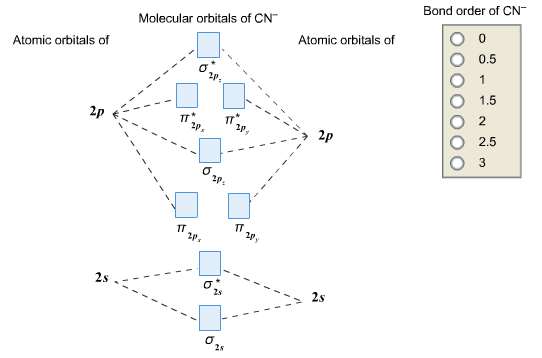# Problem: Complete this molecular orbital diagram for CN– then determine the bond order. Note that the 1s orbital is not shown in this problem.

🤓 Based on our data, we think this question is relevant for Professor MacPhail's class at DUKE.

###### FREE Expert Solution

We’re being asked to complete the molecular orbital diagram of CN- and then determine the bond order.

To do so, we shall follow these steps:

Step 1: Calculate the total valence electrons present.
Step 2: Fill the molecular orbitals with electrons.

Step 3: Determine the bond order.

Step 1: Calculate the total valence electrons present.

Group             Valence Electrons
C         4A                   1 x 4 e- = 4 e-
N         5A                   1 x 5 e- = 5 e-

From -1 charge:                       +1 e-

__________________________________
Total: 10 valence e-

Step 2Fill the molecular orbitals with electrons.###### Problem Details

Complete this molecular orbital diagram for CN then determine the bond order. Note that the 1s orbital is not shown in this problem.